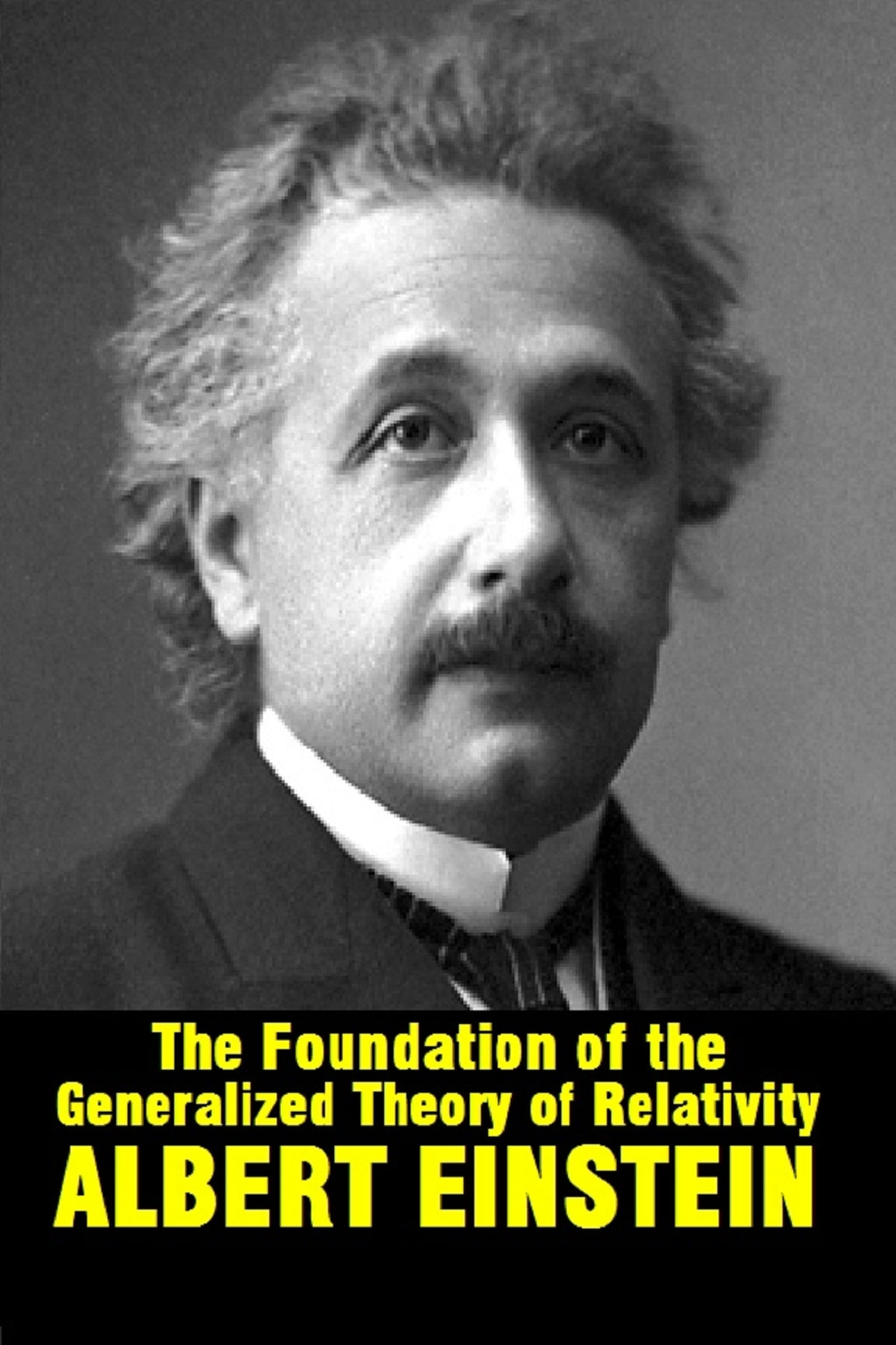# The foundation of the generalized theory of relativity- Albert Einstein

51 Seiten## Zusammenfassung

The theory which is sketched in the following pages forms the most wide-going generalization conceivable of what is at present known as "the theory of Relativity;" this latter theory I differentiate from the former "Special Relativity theory," and suppose it to be known. The generalization of the Relativity theory has been made much easier through the form given to the special Relativity theory by Minkowski, which mathematician was the first to recognize clearly the formal equivalence of the space like and time-like co-ordinates, and who made use of it in the building up of the theory. The mathematical apparatus useful for the general relativity theory, lay already complete in the "Absolute Differential Calculus", which were based on the researches of Gauss, Riemann and Christoffel on the non-Euclidean manifold, and which have been shaped into a system by Ricci and Levi-Civita, and already applied to the problems of theoretical physics. I have in part B of this communication developed in the simplest and clearest manner, all the supposed mathematical auxiliaries, not known to Physicists, which will be useful for our purpose, so that, a study of the mathematical literature is not necessary for an understanding of this paper. Finally in this place I thank my friend Grossmann, by whose help I was not only spared the study of the mathematical literature pertinent to this subject, but who also aided me in the researches on the field equations of gravitation.
Autor*innen:
Format:
E-Book
Seiten E-Book:
51
Kategorien:
Sprache:
Englisch
Erscheinungsdatum:
1.8.2020
Verlag E-Book:
Balungi Francis
ISBN E-Book:
9788835871996